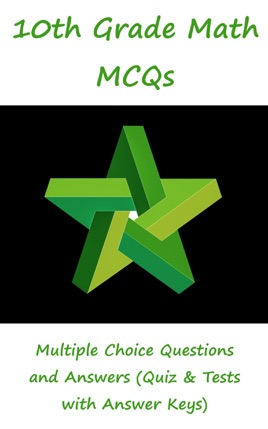• \$4.99

## Publisher Description

"10th Grade Math MCQs: Multiple Choice Questions and Answers (Quiz & Tests with Answer Keys)" provides mock tests for competitive exams preparation. "Grade 10 Math MCQ" PDF to download helps with conceptual and analytical study for self-assessment, career tests. 10th Grade Math Quizzes, a quick study guide can help to learn and practice questions for placement test preparation.

Grade 10 Math Multiple Choice Questions and Answers PDF to download is a revision guide with a collection of trivia questions to fun quiz questions and answers PDF on topics: Introduction to trigonometry, 3rd, 4th, mean and continued proportion, algebraic formulas and applications, algebraic manipulation, basic statistics, factorization, geometry, linear equations and inequalities, matrices and determinants, ratio, proportions and variations, roots of quadratic equations, sets and functions for learning.

Grade 10 Math Quiz Questions and Answers PDF also covers the syllabus of many competitive papers for admission exams of different schools from math textbooks on chapters:

3rd, 4th, Mean and Continued Proportion MCQs: 9 Multiple Choice Questions. Algebraic Formulas and Applications MCQs: 24 Multiple Choice Questions. Algebraic Manipulation MCQs: 13 Multiple Choice Questions. Basic Statistics MCQs: 28 Multiple Choice Questions. Factorization MCQs: 7 Multiple Choice Questions. General Math Questions MCQs: 26 Multiple Choice Questions. Geometry MCQs: 42 Multiple Choice Questions. Introduction to Trigonometry MCQs: 41 Multiple Choice Questions. Linear Equations and Inequalities MCQs: 25 Multiple Choice Questions. Matrices and Determinants MCQs: 47 Multiple Choice Questions. Ratio, Proportions and Variations MCQs: 21 Multiple Choice Questions. Roots of Quadratic Equations MCQs: 24 Multiple Choice Questions. Sets and Functions MCQs: 24 Multiple Choice Questions.

3rd, 4th, Mean and Continued Proportion MCQs PDF: It covers quiz questions about continued proportional, fourth proportional, mean proportional, and third proportional.

Algebraic Formulas and Applications MCQs PDF: It covers quiz questions about algebraic expressions, math formulas, surds and surds applications.

Basic Statistics MCQs PDF: It covers quiz questions about construction of frequency polygon, construction of histograms, frequency distribution, measures of central tendency, and measures of dispersion.

Factorization MCQs PDF: It covers quiz questions about factorization of expressions, and math theorems.

General Math Questions MCQs PDF: It covers quiz questions about basic concepts, circle's basic concepts, fraction, improper and proper fraction, rational fraction, math theorems, parallel lines, roots, and coefficients.

Geometry MCQs PDF: It covers quiz questions about circles, radius of circle, escribed circle, cylinder, lines and angles, polygon, rectangle, and triangle.

Introduction to Trigonometry MCQs PDF: It covers quiz questions about trigonometric identities, trigonometric ratios, area of circular sector, co terminal angles, conversion: radians and degrees, degree, measurement of angles, quadrants, radian measure of angle, reciprocal identities, relation between radians and degree, and sector of circle.

Matrices and Determinants MCQs PDF: It covers quiz questions about introduction to matrices, types of matrices, addition and subtraction of matrices, multiplication of matrices, multiplicative inverse of matrix, and solution of simultaneous linear equations.

Ratio, Proportions and Variations MCQs PDF: It covers quiz questions about ratios, proportion, variation, joint variation, k method, and math theorems.

Roots of Quadratic Equations MCQs PDF: It covers quiz questions about quadratic equation, solution of quadratic equations complex cube roots of unity, discriminant, radical equation, and reciprocal equation.

Sets and Functions MCQs PDF: It covers quiz questions about sets, recognize of operations on sets, example of sets, binary relation, and ordered pairs.